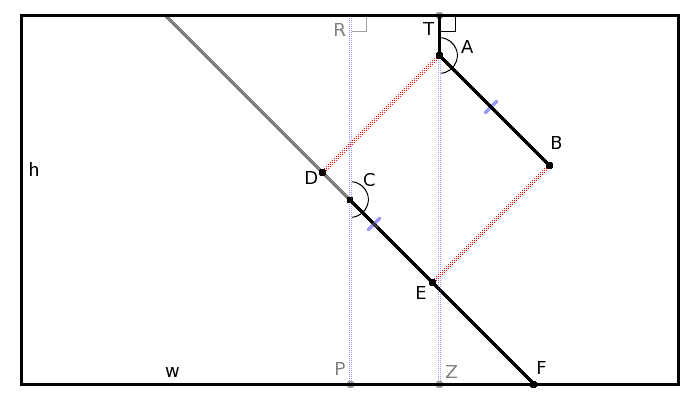# Find Point On Rect Edge

Overview

I've been struggling at this problem for about 3 days now. I've posted it on a couple forums will no answers, so maybe I'll get a solution here. Thanks in advance!Plain Diagram: http://www.swivelgames.com/projects/ideas/circlepoints/getseglength_simpler.png

Known: A, B, C, P, R, T, Z, h, w

Need: D, E, F; Length of dcef and ef segments

Y axis is inverted!!

(0,0) is at top left corner of rectangle

Known Data
h = 600
w = 800

C is at (w/2,h2) = (400,300)
R is at (400,0)
P is at (400,600)

A is at (534,88)
B is at (740,296)
T is at (534,0)
Z is at (534,600)

∠TAB = 135°
∠ZAB = 45°

∠RCE = ∠TAB
∠PCE = ∠ZAB

Problem Solving
∠ZFE = 90 - ∠PCE
∠ZFE = 45°

segCP = segRC = 400
segCF = sin(∠PCF) × segCP
segCF = sin(45) × 400
segCF = 282.8427125

The Question!

Now all I really need is the length of segEF to solve the problem, since we know that segDE = segAB.

So segDF = segDE + segEF

Can anyone help me get segEF?

Something to note: This is a real-world problem where ∠TAB will be anywhere from 0° to 360°, which in-turn would affect what side and position F would be. And ∠RCF always = ∠TAB